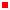Bionics Lab › Education > Classes > MAE 263B - Dynamics of Robotic Systems

# Classes

 Instructor Jacob Rosen Office: Engineering IV Building, Room 37-146 Voice Office: 831.459.5302 e-mail: jacobrosen@ucla.edu Anonymous Email (For the Subject Line use: MAE 263B) Office Hours: Wed 12:00-2:00 Signup Sheet - (Entry MAE163B/263B - ) Zoom (Presonal Link) TA Mianzhi Zhou Email: zhoumianzhi@ucla.edu MAE 263BDynamics of Robotic Systems Course Summary: Motion planning and control of articulated dynamic systems: nonlinear joint control, experiments in joint control and multiaxis coordination, multibody dynamics, trajectory planning, motion optimization, dynamic performance and manipulator design, kinematic redundancies, motion planning of manipulators in space, obstacle avoidance. Recommended preparation: courses 155, 171A, 263A, Assignments & Grading: HW Assignments - Problem Sets 20% Paper Review (HW 0) 5% Mid Term Exam (Take Home) 30% Final Exam (Take Home) 40% Participation 5% Textbook: (*) John Craig, Introduction to Robotics: Mechanism & Control, 4ed Edition, Addison Wesley 2018 (*) Lung-Wen Tsai, Robotic Analysis, Wiley 1999, Ch. 9, Dynamics of Serial Manipulators (*) Matjaž Mihelj, Tadej Bajd, Aleš Ude, Jadran Lenarčič, Aleš Stanovnik, Marko Munih, Jure Rejc, Sebastjan Šlajpah, Robotics, Springer 2019 Chapter 10: Robot Control Class Notes Introduction Class 00: Class Introduction Class 01: Introduction & Basic Ideas Direct & Inverse Kinematics (Review) Class 02: Special Description & Transformation (Review) Class Supplement: Equivalent angle axis vector Class 03: Direct Manipulator Kinematics (Review) Class 04: Inverse Manipulator Kinematics (Review) Trajectory Generation Class 04: Trajectory Generation (1/2) Class 05: Trajectory Generation (2/2) Jacobian Class 06: Advanced Kinematics (Velocities - Linear & Angular) Class 07: Jacobian - Introduction Class 08: Jacobian - Velocity Propagation Method - Part 1 Class 09: Jacobian - Velocity Propagation Method - Part 2 Class 10: Jacobian - Force Propagation Method Class 11: Jacobian - Explicit Method Class 12: Jacobian - Implications & Applications Dynamics Class 13: Dynamics - Newton-Euler Eqautions - Theory (1/4) Class 14: Dynamics - Tensor of Inertia (2/4) Class 15: Dynamics - Newton-Euler Eqautions - 2R Example (3/4) Class 16: Dynamics - Lagrange Equations - Theory & 2R Example (4/4) Control Class 17: Control Design - PID Design Class 18: Control Design - Bode Design (Lead/Lag) Class 19: Control Design - Nyquest Design (Gain/Phase Margins) Class 20 : Intro to Control Systems - Control Scheme 1 Class 21 : Intro to Control Systems - Control Scheme 2 Section Notes (TA) Section 01: Forward & Inverse Kinematics (Yasukawa Motoman L-3) Section 02: Robotics - Matlab ToolBox (Matlab Example - XML ) Section 03: Jacobian - Velocity Propagation Direct Diff (SCARA) Section 04: Jacobian - Force Propagation (SCARA) Section 05: x Section 06: x Section 07: x Section 08: x Section 09: x Projects - Homework   Labs (Denso Robotic Arms) Exams (Take Home) Midterm Exam - Take Home - Due Week 6 (Friday Midnigh) Final Exam - Take Home - Due Week 12 (Friday Midnight) Matlab Robotic Toolbox - Free (by Corke) Robotics Toolbox (Release 10) Introduction to Robotics Toolbox for MATLAB (Powerpoint Slides - TA) The book Robotics, Vision & Control, Second Edition (Corke, 2017) is a detailed introduction to mobile robotics, navigation, localization; and arm robot kinematics, Jacobians and dynamics illustrated using the Robotics Toolbox for MATLAB (Availble on-line to UCLA Students) Matlab Code (Robotic Toolbox) Puma 560 (Modified DH) - StdMod_puma560.m Robotic Toolbox (by Mathworks)   Matlab Demo Mathematica Demos (Robotics) Note: A viewer is required for running the demos off line Special Description & Transformation Randomize Motion for Six Degrees of Freedom Mathematica Demo Robot Manipulator Workspaces YouTube Explanation Mathematica Demo Denavit-Hartenberg Parameters for a Three-Link Robot Mathematica Demo Common Robot Arm Configurations Mathematica Demo Three Parametrizations of Rotations Configurations YouTube Explanation Mathematica Demo Forward and Inverse Kinematics Model of an Industrial Robot Arm Mathematica Demo Inverse Kinematics for a Robot Manipulator with 6 DOF Mathematica Demo Forward Kinematics Mathematica Demo Inverse Kinematics Mathematica Demo Forward and Inverse Kinematics of the SCARA Robot Mathematica Demo A Model of the SCARA Robott Mathematica Demo Kinematics of SCARA Robot in 2D Mathematica Demo Kinematics of a Redundant Anthropomorphic Arm with 7 DOF Mathematica Demo Inverse Kinematics in Redundant Robot Manipulator (Swivel Angle) YouTube Explanation Mathematica Demo Manipulability Forward and Inverse Kinematics for Two-Link Arm Mathematica Demo Manipulability Ellipsoid of a Robot Arm Mathematica Demo Trajectory Planning Trajectory Planning of Robot for Painting Art Mathematica Demo   Autolev Industrial Robotic Arms - Companies Notes (*) CAD files are available on-line (+) Data sheet / Specs / Schematic drawings are available on-line Videos (YouTube) General Video DH Parameters Denavit-Hartenberg Reference Frame Layout